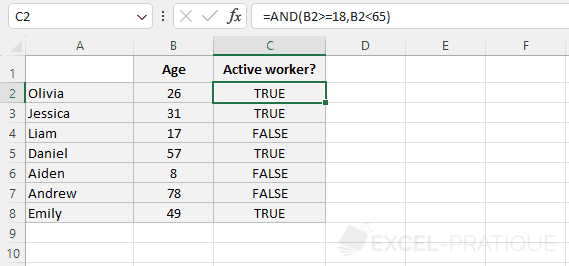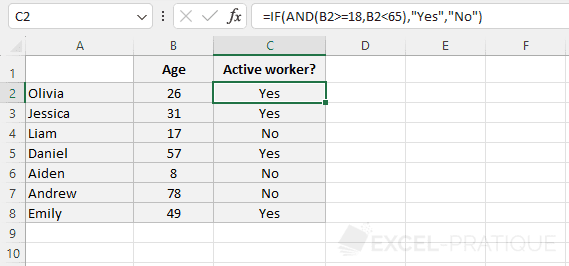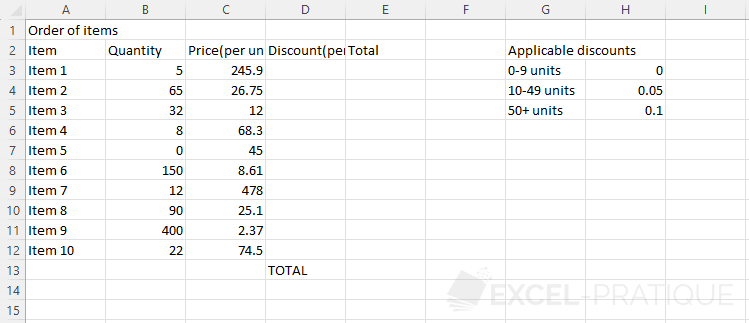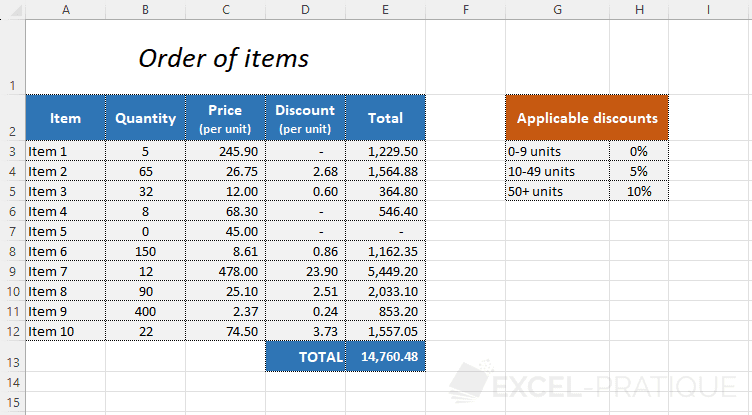# Excel Training: Comparison Operators

In the examples of the IF function, you have seen a first comparison operator `>=`. Here are the other operators that you can use in your formulas:

 = is equal to <> is different than < is less than <= is less than or equal to > is greater than >= is greater than or equal to

## The AND and OR functions

The AND function `=AND(test_1, test_2, ...)` returns TRUE if all the tests are TRUE.

The OR function `=OR(test_1, test_2, ...)` returns TRUE if at least one of the tests is TRUE.

For example, to display TRUE when the person is at least 18 years old AND less than 65 years old (and FALSE otherwise), enter `=AND(B2>=18,B2<65)`:The AND and OR functions can only return the values TRUE or FALSE.

In this case, to get values other than TRUE or FALSE, nest the AND function in an IF function instead of the logical test (this will work perfectly because the logical test seeks to determine whether the result is TRUE or FALSE).

The formula then becomes `=IF(AND(B2>=18,B2<65),"Yes","No")`:## More functions

You will find examples of using the most common functions on the page dedicated to functions (accessible via the "Excel Functions" link in the menu at the top of the page).

## Exercise

To put this lesson on formulas and functions into practice, download the following exercise: exercise-4.xlsx